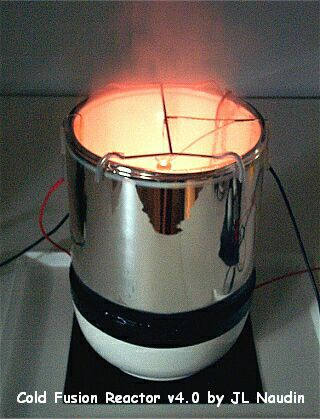Full tests of the CFR v4.0
created on May 8, 2003 - JLN Labs - Last update June 13, 2003
Toutes les informations et schémas sont publiés gratuitement ( freeware ) et sont destinés à un usage personnel et non commercial
All informations and diagrams are published freely (freeware) and are intended for a private use and a non commercial use.

The purpose of this serie of tests is to find the working regime of the Cold Fusion Reactor ( CFR ) v4.0. So, different voltages values have been tested and also " a blank test " have been conducted with the reactor set at a cold glow discharge plasma regime. This " blank test " has been done at low voltage and under the normal working regime of the CFR which is a high temperature plasma and an incandescent tungsten cathode.

RUN#29 - " BLANK TEST " : Low Power test in the cold glow discharge plasma regime ( 155V DC ) :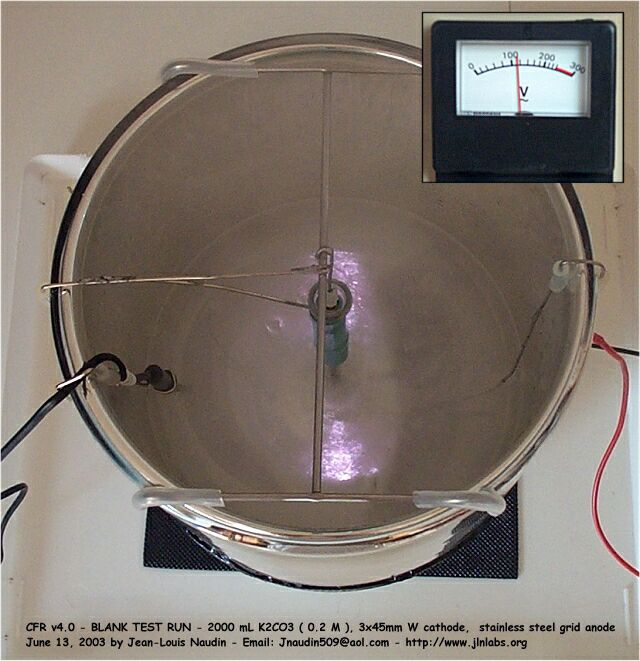For the "Blank test", the Voltage input has been tuned so as to get a cold glow discharge plasma around the W cathode.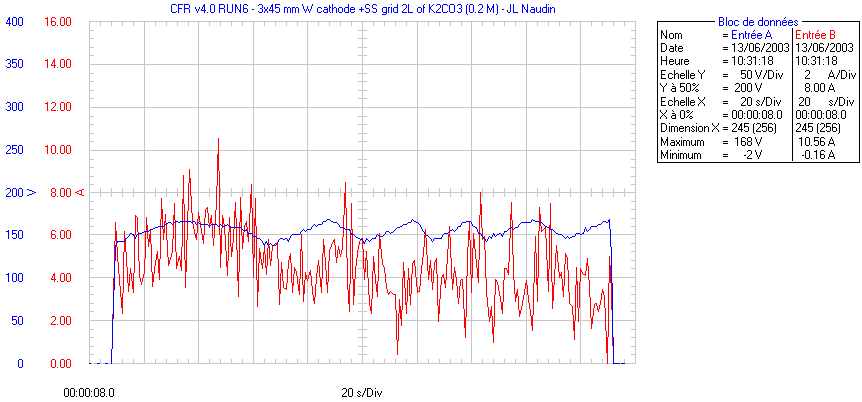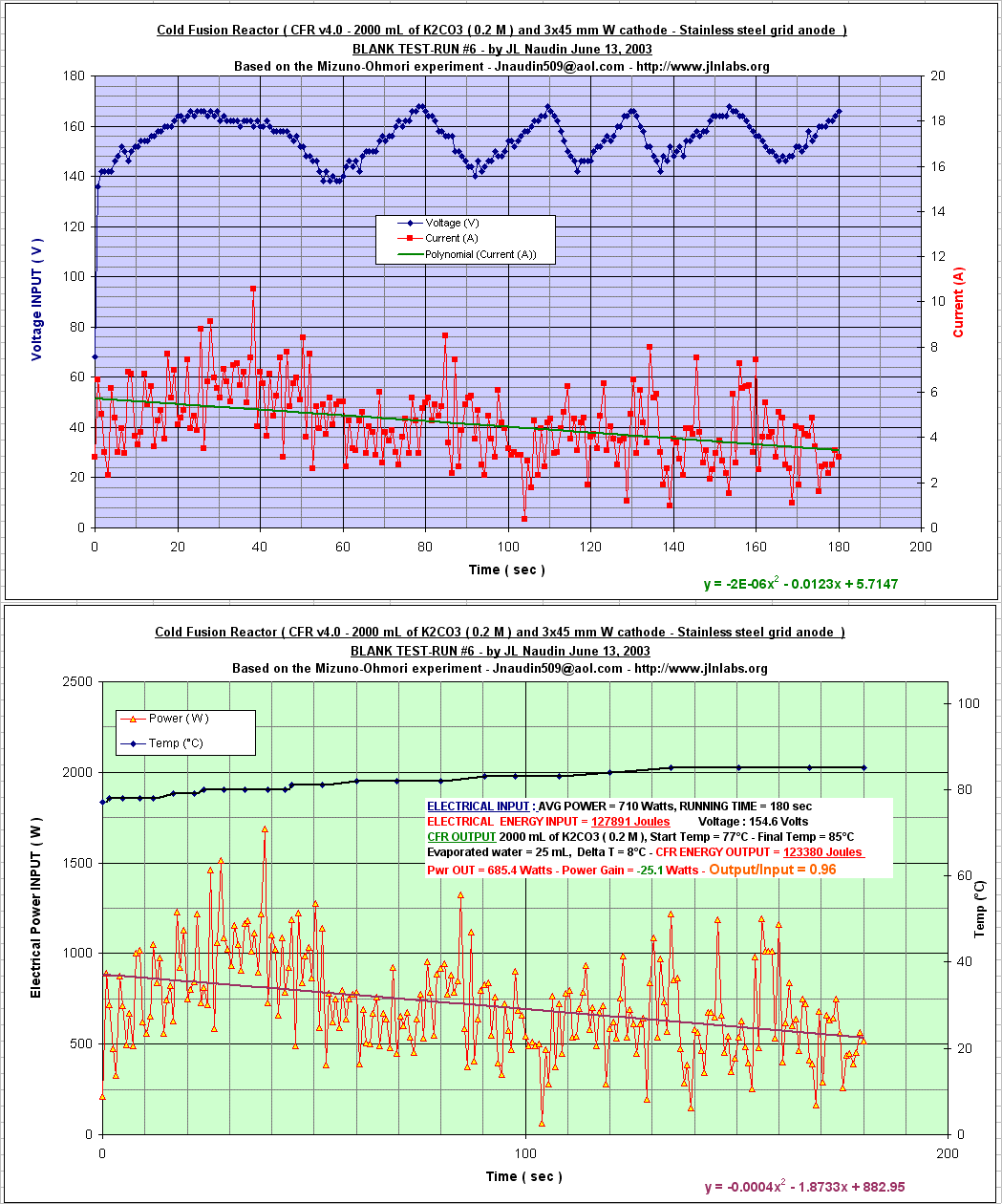Electrolyte solution : 2000 mL of K2CO3 ( 0.2 M ), ELECTRICAL INPUT : AVG POWER = 710 Watts, Voltage : 154.6 Volts RUNNING TIME = 180 sec ELECTRICAL ENERGY INPUT = 127891 Joules CFR OUTPUT : Start Temp = 77°C - Final Temp = 85°C Evaporated water = 25 mL, Delta T = 8°C - CFR ENERGY OUTPUT = 123380 Joules Pwr OUT = 685.4 Watts - Power Gain = -25.1 Watts BLANK TEST : COP = Output/Input = 0.96

RUN #26: Test at 230V DC :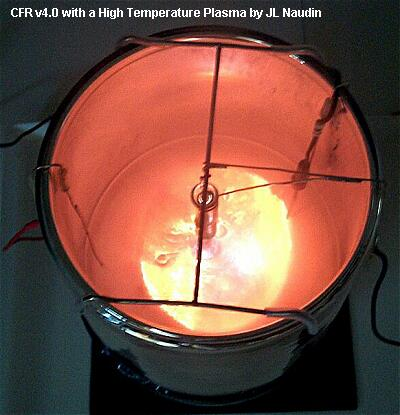In the full regime there is a Hot and strong plasma around the W cathode.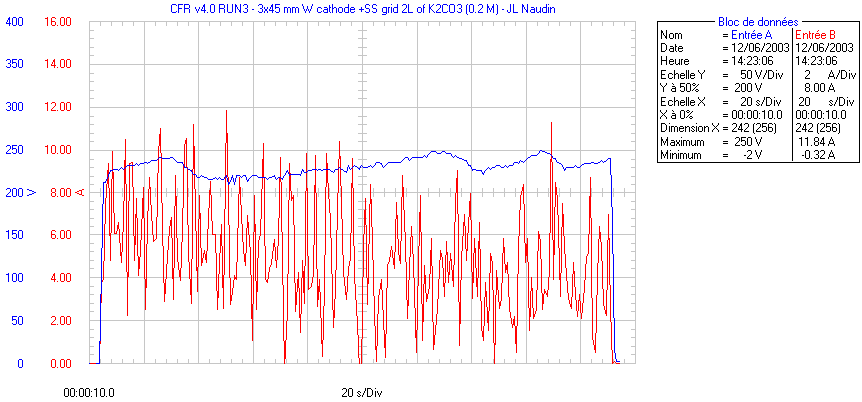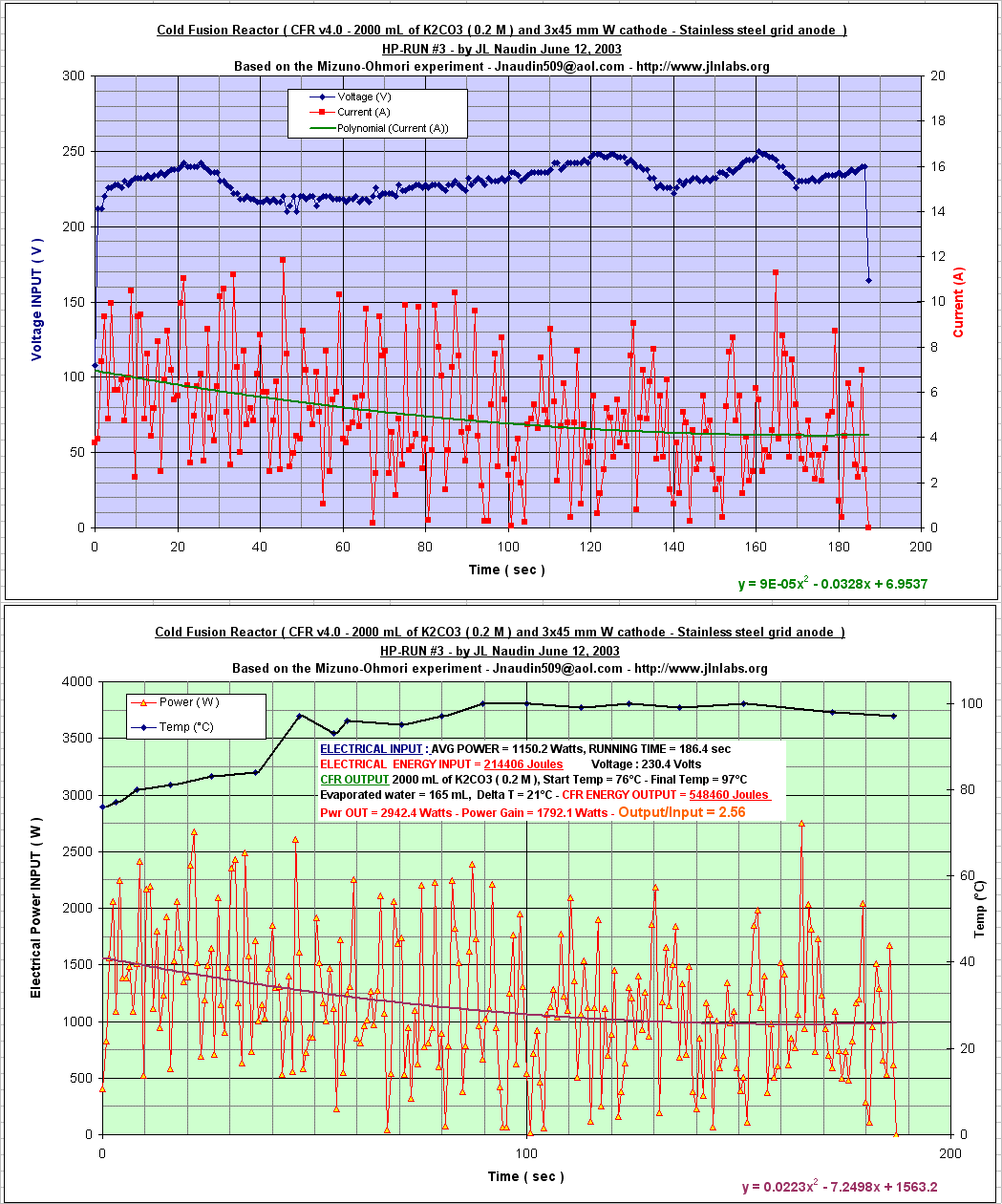Electrolyte solution : 2000 mL of K2CO3 ( 0.2 M ), ELECTRICAL INPUT : AVG POWER = 1150.2 Watts, Voltage : 230.4 Volts RUNNING TIME = 186.4 sec ELECTRICAL ENERGY INPUT = 214406 Joules CFR OUTPUT : Start Temp = 76°C - Final Temp = 97°C Evaporated water = 165 mL, Delta T = 21°C CFR ENERGY OUTPUT = 548460 Joules Pwr OUT = 2942.4 Watts - Power Gain = 1792.1 Watts COP = Output/Input = 2.56

RUN #27: Test at 231V DC :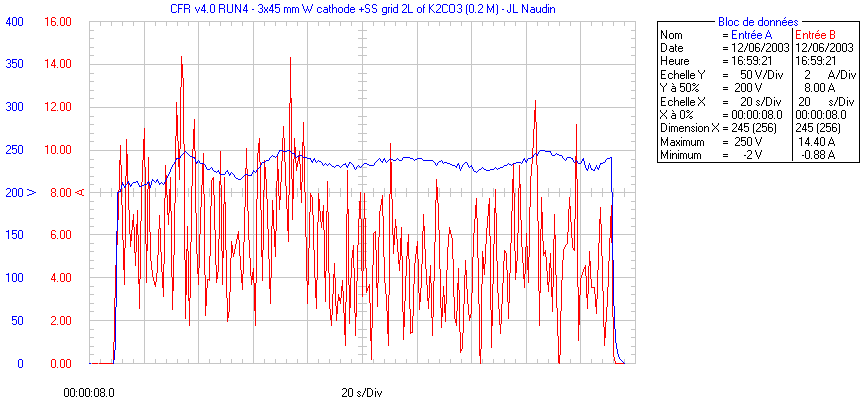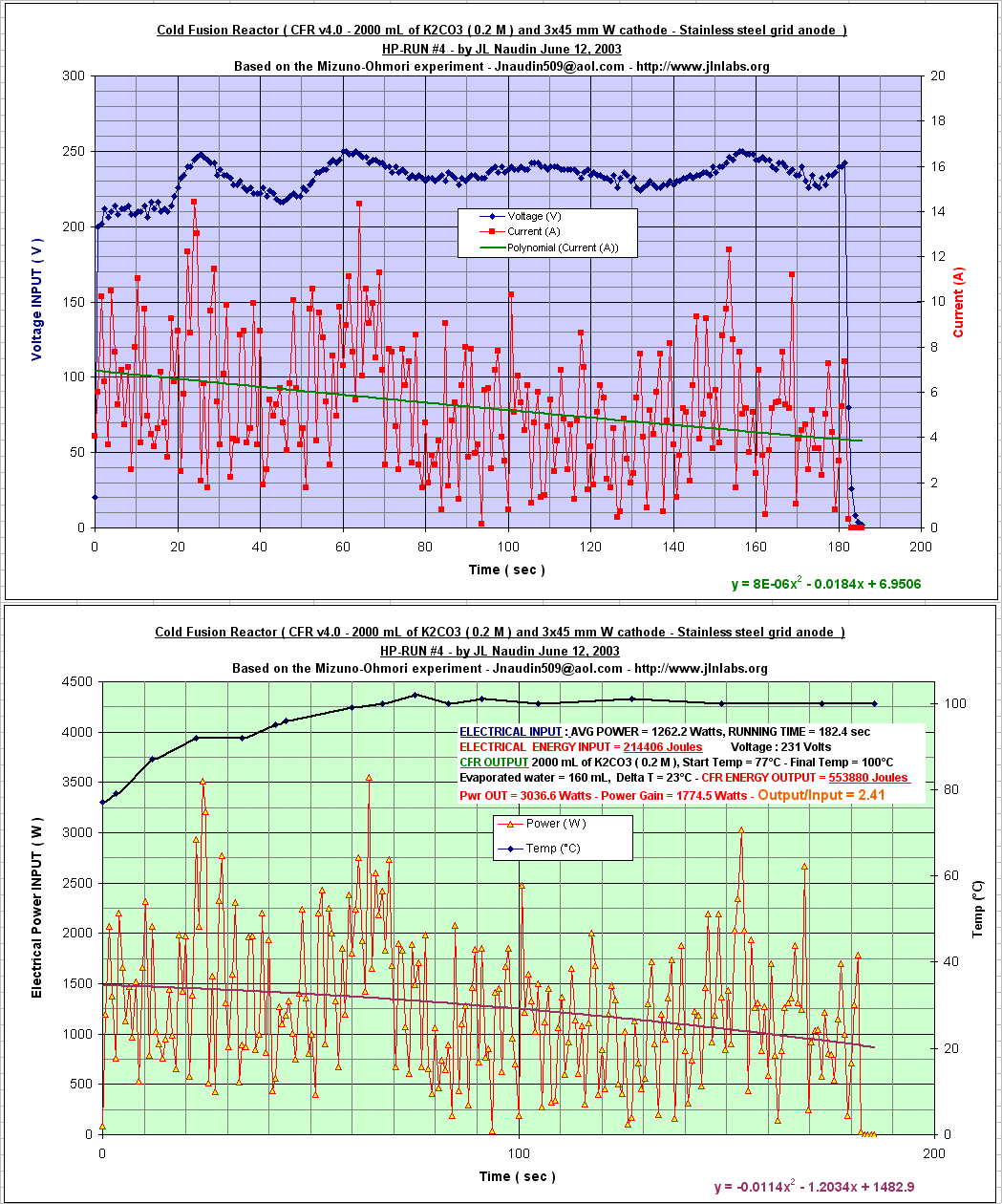Electrolyte solution : 2000 mL of K2CO3 ( 0.2 M ), ELECTRICAL INPUT : AVG POWER = 1262.2 Watts, Voltage : 231 Volts RUNNING TIME = 182.4 sec ELECTRICAL ENERGY INPUT = 214406 Joules CFR OUTPUT : Start Temp = 77°C - Final Temp = 100°C Evaporated water = 160 mL, Delta T = 23°C CFR ENERGY OUTPUT = 553880 Joules Pwr OUT = 3036.6 Watts - Power Gain = 1774.5 Watts COP = Output/Input = 2.41

RUN #28: Test at 233V DC :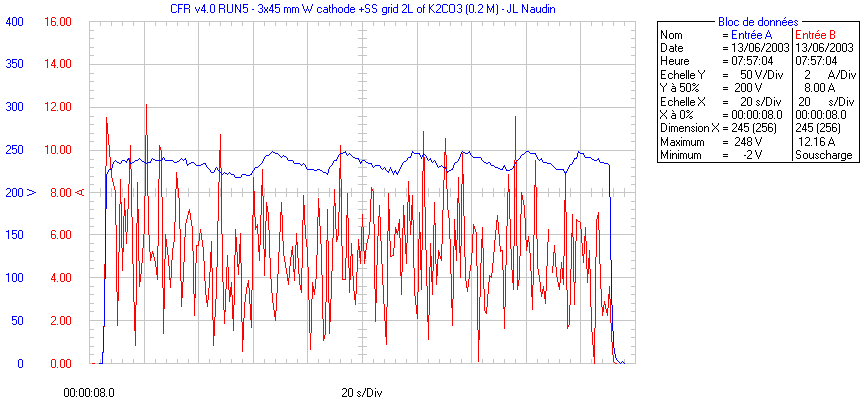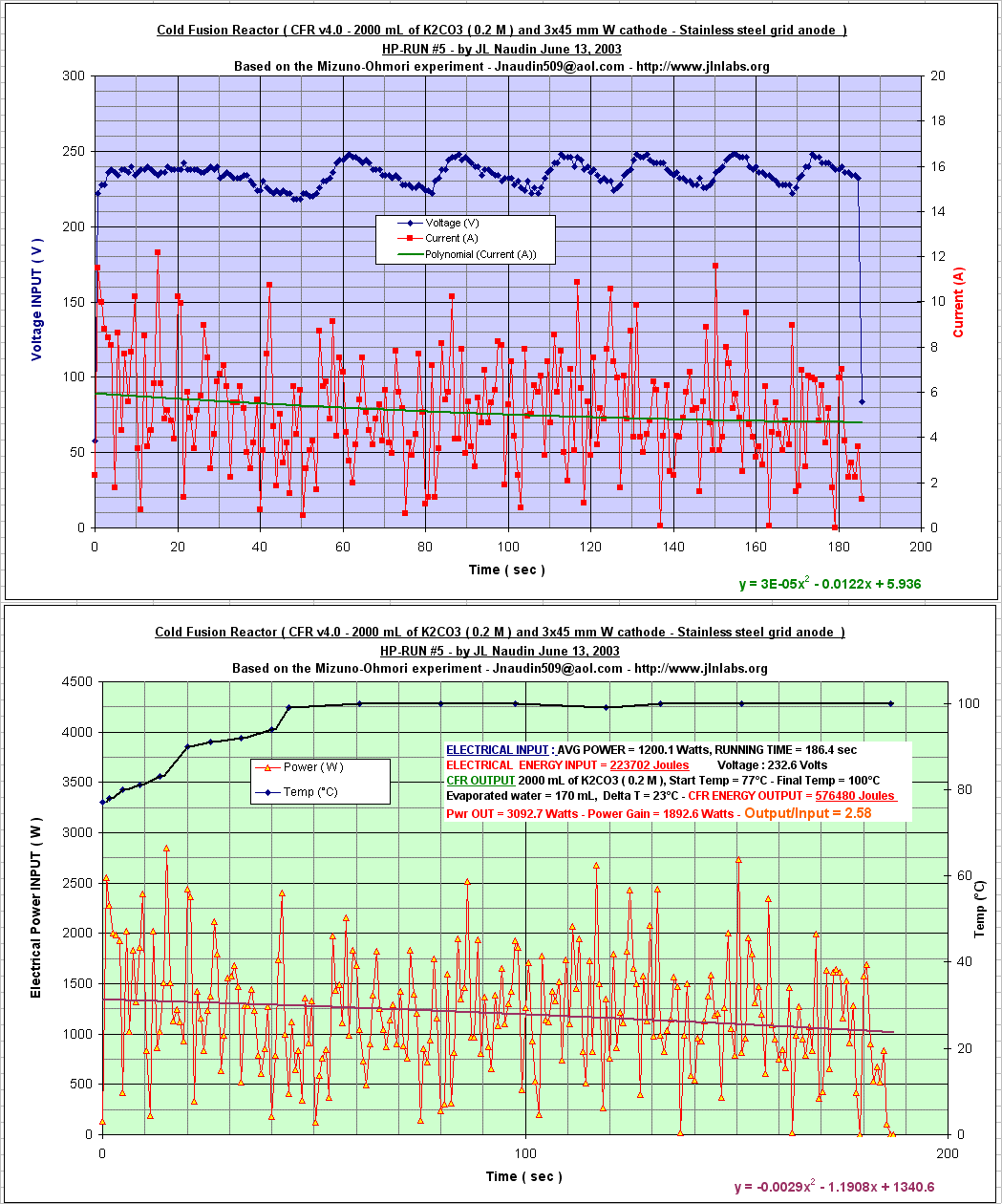Electrolyte solution : 2000 mL of K2CO3 ( 0.2 M ), ELECTRICAL INPUT : AVG POWER = 1200.1 Watts, Voltage : 232.6 Volts RUNNING TIME = 186.4 sec ELECTRICAL ENERGY INPUT = 223702 Joules CFR OUTPUT : Start Temp = 77°C - Final Temp = 100°C Evaporated water = 170 mL, Delta T = 23°C CFR ENERGY OUTPUT = 576480 Joules Pwr OUT = 3092.7 Watts - Power Gain = 1892.6 Watts COP = Output/Input = 2.58

The results of the TESTS RUNS #24 and #25 are published here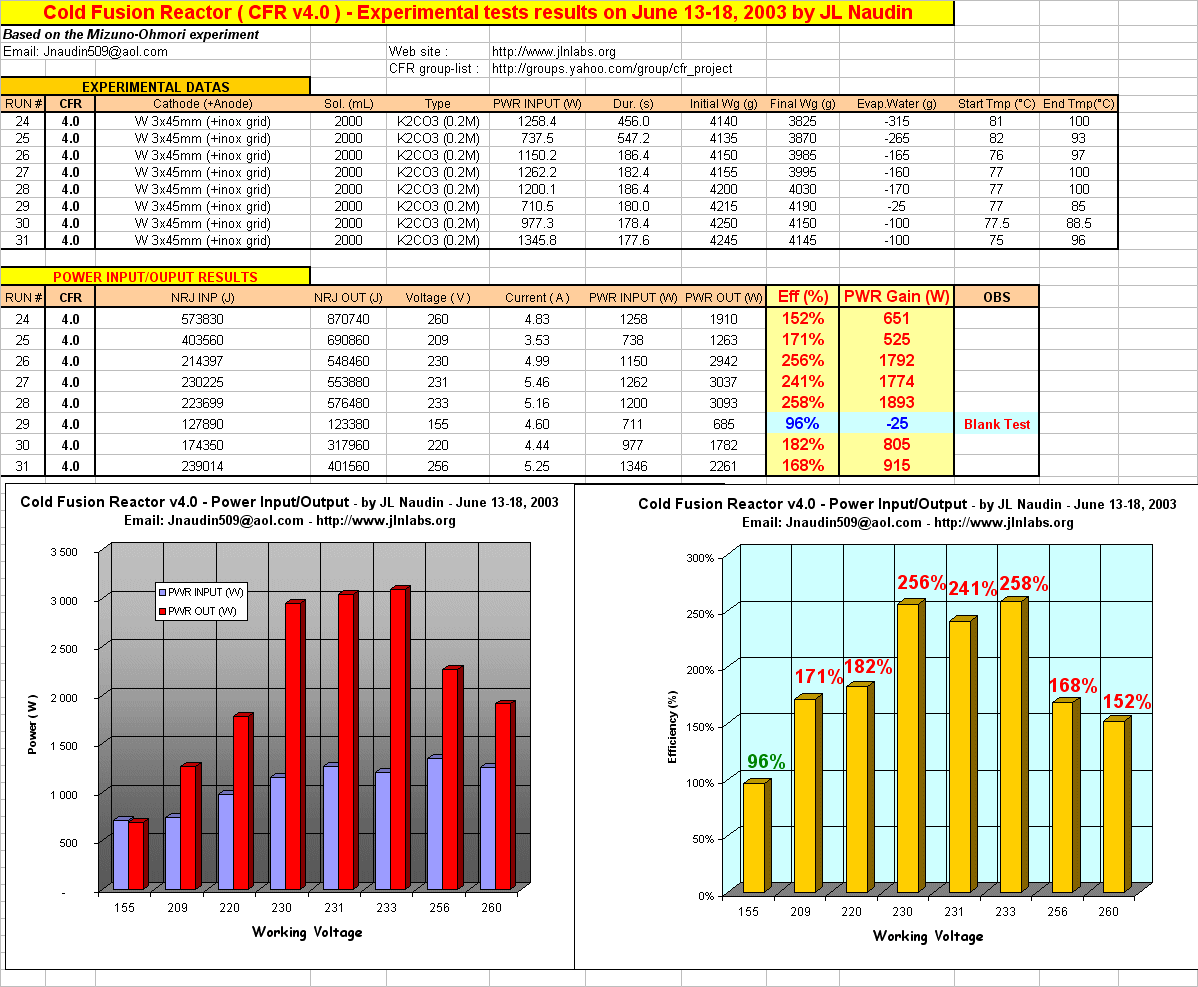Notes from Jean-Louis Naudin : This serie of tests on the CFR v4.0, shows that the maximum of efficiency ( about 250% ) with a such setup is obtained for a voltage about 230 V DC. At low voltage ( < 160 V DC ) the reactor efficiency is under 100% ( see the "Blank test" ) even if a glow discharge plasma can be seen around the Tungsten cathode. A High Temperature plasma with an incandescent cathode is required to get the effect. With too much voltage >250V DC and thus too much power, the measured efficiency begins to drop due to the recondensation effect of the steam produced at the output of the reactor.

I am very grateful to the Professor Pierre Clauzon for his contribution to the CFR project and his help during some CFR tests runs and also to the Professor Jean-François Fauvarque and Gérard Lallevé from the "Laboratoire d'Electrochimie Industrielle" of the CNAM ( Conservatoire National des Arts et Métiers ) from Paris, for their scientific support and their contribution to this project.

A 30 mn tests with the CFR v4.0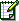Email : JNaudin509@aol.com